Courses

# Simplification MCQ 1

## 20 Questions MCQ Test Quantitative Techniques for CLAT | Simplification MCQ 1

Description
This mock test of Simplification MCQ 1 for Quant helps you for every Quant entrance exam. This contains 20 Multiple Choice Questions for Quant Simplification MCQ 1 (mcq) to study with solutions a complete question bank. The solved questions answers in this Simplification MCQ 1 quiz give you a good mix of easy questions and tough questions. Quant students definitely take this Simplification MCQ 1 exercise for a better result in the exam. You can find other Simplification MCQ 1 extra questions, long questions & short questions for Quant on EduRev as well by searching above.
QUESTION: 1

Solution:
QUESTION: 2

Solution:
QUESTION: 3

###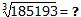Solution:
QUESTION: 4

? % of 1400 – 18% of 750 = 159

Solution:
QUESTION: 5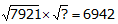Solution:
QUESTION: 6

856 x 567 = ?

Solution:
QUESTION: 7

(?)3 x (55) ÷ 225 = 3000

Solution:
QUESTION: 8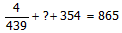Solution:
QUESTION: 9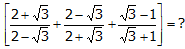Solution:
QUESTION: 10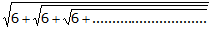Solution:

On solving, we will get x = 3 or x = -2
Ignoring the negative value answer is 3.

QUESTION: 11

82/3 is equal to

Solution:
QUESTION: 12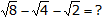Solution: √8-√4-√2=√2×4-√4-√2 =√4-√2=√2-2 ans
QUESTION: 13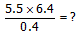Solution:

5.5 x 6.4 / 0.4 =  35.2 / 0.4 = 88

QUESTION: 14

(764 x ?) ÷ 250 = 382

Solution:
QUESTION: 15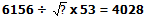Solution:

C is the correct option. Solution-
4028÷53=76
6156÷76=81
So the value needed is a square of 81 which is 6561.

QUESTION: 16

[(7 x 9) + (2 x 12) + 3] / [(12 x 3) + (81/9)] = ?

Solution:

[(7 x 9) + (2 x 12) + 3] / [(12 x 3) + (81 / 9)]
= (63 + 24 + 3) / (36 + 9)
= 90/45
= 2

QUESTION: 17

(169 – 144)½ ÷ (64 + 36)½ = ?

Solution:
QUESTION: 18

–224 + (–314) x (–9) = ?

Solution:

Option (a) 2602 is correct.

Explanation:-

{ Given,

-224 + ( - 314) * (-9)

According to BODMASS ;

=>  -224 -314  * -9

=>  -224 +  2826
=>   2602

QUESTION: 19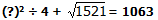Solution:
QUESTION: 20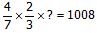Solution: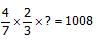8/21 * x = 1008

x = 21168 / 8

x = 2646